Home > GB8I > Chapter cc46 > Lesson cc46.3.3 > Problem6-110

6-110.
1.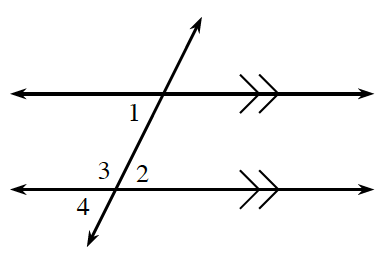6-110. Refer to the diagram at right. Homework Help ✎

1. If m∠1 = 74º and m∠4 = 3x − 18º, write an equation and solve for x.

2. If m∠2 = 3x − 9º and m∠1 = x + 25º, write an equation and solve for x. Then determine m∠2.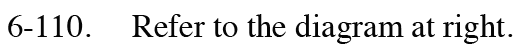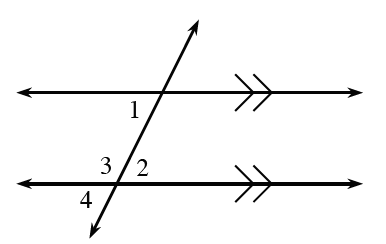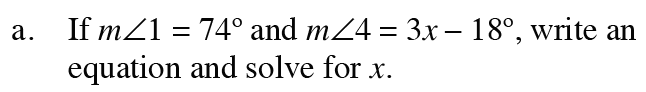3x − 18 = 74

3x = 92

x = 30.6°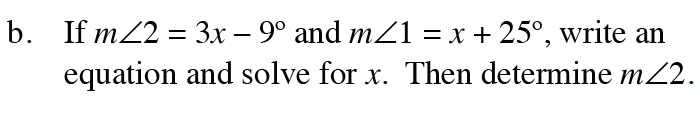Disregard your calculations for angle measure from part (a).

Since the lines are parallel, angles 1 and 2 have equal measure.

x = 17°, m∠2 = 42°